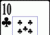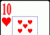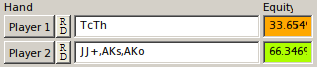# 4Betting Mathematics28 Mar, 2011

This was inspired by a friend of mine who plays NL50. She wanted to work out whether she should 4bet/call in a spot against a regular.

So here is an example of mine to explain it.

\$0.50/\$1 NL (6max) 4 handed

Hero CO (\$100)
BTN (\$100)
SB (\$100)
BB (\$100)

Preflop: Hero is the CO and is dealtHero raises to \$3, BTN folds, SB re-raises \$11, BB folds, Hero... considering whether we can 4bet/call

## The basic mathematics.

I will try to keep the following mathematics as algebra free as I can.

Our expected value (EV) in this situation can be broken down into two parts:

1. Our EV when we get all in. (i.e. we 4bet and then call any raises).
2. Our EV when our opponent folds.

To calculate the EV of 4bet/calling, you first need to work out the EV for the following two situations:

``````EV when all in = ( [total pot - what we risk] * our equity) - (what we risk * opponent's equity)
``````
``````EV when opponent folds = we win whatever is in the pot
``````

We can then combine these two EV equations together to get the Total EV for our 4bet/call:

``````Total EV = (EV when all in * how often we get it in) + (EV when opponent folds * how often opponent folds)
``````

## Ranges.

Let's think about our opponent's range, and assume they are going to be shoving over our 4bet with JJ+ and AK. PokerStove tells us that our equity in the hand against this range is `33.6%`.We are at an equity disadvantage when we get called.

We also know that if we commit to 4bet/calling, we will be risking `97bb` (we put in `3bb` already) to win a total pot of `201bb` (both of our stacks plus the big blind).

Therefore, the EV of getting the stacks in is:

``````EV when all in = (201-97 * 0.336) - (97 * 0.634)
EV when all in = 34.9 - 61.5
EV when all in = -26.6bb
``````

So clearly we have a negative expectation when we get all the money in, and that is because we are getting the money in with `33.6%` equity vs their range. However, "EV when all in" is only part of the equation – we also have to consider how much we win when our opponent folds too.

When our opponent folds after we 4bet, we win `15bb` (their `11bb`, plus the `1bb` and our `3bb` initial raise). Therefore:

``````EV when opponent folds = +15bb
``````

## Fold equity.

There must be a cutoff point where the small blind folds to our 4bet so often that it doesn't matter if we lose money when we get all in, because we more than make up for it the times they fold. This is called fold equity.

We can construct a simple equation to find out whether we have any fold equity. All we need to do is replace how often we need them to fold with `x`, and set the answer of the equation to `0`. Then we can solve the equation for `x`, and it will tell us how often our opponent must fold in order to break even.

It is basically a re-arrangement of the formula for Total EV of the play:

``````Fold Equity:

0 = [what we win when opponent folds * x] - [what we lose when opponent doesn't fold * (1-x)]
``````

From the figures we've already calculated, we know that when our opponent folds we win `+15bb`, and when they do not fold we lose `26.6bb` (because we get all the money in).

Therefore we can substitute the numbers into the equation, solve it, and get a figure for `x`:

``````Fold Equity:

0 = [15 * x] - [26.6 * (1-x)]
0 = 15x - 26.6 + 26.6x
0 = 41.6x - 26.6
26.6 = 41.6x
x = 26.6/41.6
x = 0.64, or 64%.
``````
In other words, our opponent needs to fold more than 64% of the time for our 4bet to be profitable.

To put this into more useful terms:

• If our opponent is 3betting `8%` or less, then we make a loss.
• If our opponent is 3betting `9%` of hands in this spot and shoving the top 3% of hands (`JJ+` and `AK`), we show a profit by 4bet/calling `TT` here.
• If our opponent is 3betting `10%` or more, then we are just printing money by 4betting.

It is impossible to model whether 4betting is better than calling, but you don't want to spend a ton of time focusing on these type of spots – once you know whether 4betting is +EV or not, you can just go from there.

## Conclusion.

You should spend a decent amount of time going back over this article and re-entering numbers based on different shoving ranges and different starting hands for yourself. For example:

• What happens when our opponent is 5bet bluffing sometimes?
• What if if they are only shoving `QQ+`?
• What if we have `AQ` instead of `TT`?

Jack.